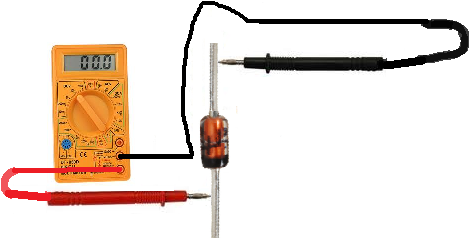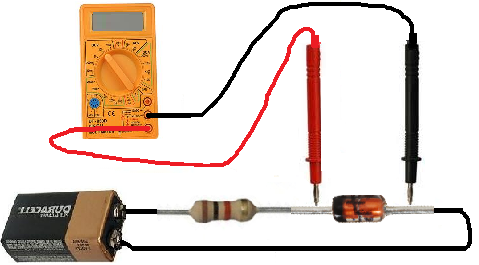﻿ How to Test a Zener Diode# How to Test a Zener DiodeIf you want to check a zener diode is whether it is good or defective, there are two simple but effective quick tests that you can do.

We will discuss both tests in this article.

### Test a Zener Diode with an Ohmmeter of a Multimeter

A very good test you can do is to check a zener diode with your multimeter set to the ohmmeter setting.

This is a simple test we can do to check whether it is good, open, or shorted.

So we take the ohmmeter and place it across the leads of the diode. The orientation is very important.

### Anode-Cathode Diode Resistance Test

We first take the ohmmeter and place the positive probe on the anode of the diode (the lead attached to the brown part of the zener diode) and the negative probe on the cathode of the diode (the lead connected on the side of the zener diode with the black strip), as shown above. In this setup, the diode should read a moderately low resistance, maybe a few hundreds of thousands of ohms. For example, you may read around 450KΩ.### Cathode-Anode Diode Resistance Test

Now take the ohmmeter and switch the probes around so that the positive probe of the multimeter is now on the cathode of the diode and the negative probe is on the anode of the diode. In this setup now, the diode should read a much higher resistance, well over 1MΩ. A typical reading may, for example, be 2.3MΩ. The multimeter may even indicate 'OL' for an open circuit, since the resistance is so high.If you read a moderately low resistance with the leads on the zener diode one way and a high resistance with the leads the other, this is a sign that the zener diode is good. A zener diode should read relatively low resistance in the forward biased direction and very high resistance in the reverse biased direction.

### Open Diode

If a zener diode reads high resistance in both directions, this is a sign that the diode is open. A diode should not measure very high resistance in the forward biased direction. The diode should be replaced in the circuit.

### Shorted Diode

If the zener diode reads low resistances in both directions, this is a sign that the diode is shorted. A diode should not measure low resistance in the reverse biased direction. The diode should be replaced in the circuit.

### How to Test a Zener Diode with a Voltmeter of a Multimeter

A second test you can do to check to see whether a zener diode is defective or not is to measure its voltage with a voltmeter of a multimeter (or simply just a voltmeter if you have one.)

In order for this test to work, we must feed voltage to the zener diode in reverse bias in series with a resistor. The voltage must be higher than the rated zener voltage of the zener diode. In the circuit below, we feed the zener diode and a 1KΩ resistor 9 volts from a 9-volt battery. The zener diode is rated for 5.1V, well below the 9 volts supplied. When measuring the voltage across the zener diode, it must measure a voltage near its rated zener voltage.If a zener diode reads a voltage near its rated zener voltage, VZ, then it is good.

### Open Diode

If the diode reads a much higher voltage, of or near the voltage of the supply voltage feeding it, it is open and, thus, defective. It should be replaced.

### Shorted Diode

If the diode is reading a much lower voltage than its rated voltage, such as near 0V, it is shorted internally and, thus, is defective and should be replaced.

These are 2 simple but effective tests to test a zener diode.

HTML Comment Box is loading comments...